# Filter or Remove Columns

Assume that in MS Excel, you have a table consisting of a few columns consisting of few values, and you want to filter to remove the specified columns from the table. You might take it easy and would prefer to manually filter out to remove the desired columns from the table, without any need for the formula; then congratulations because you are thinking right.

But let me add that it would be a big deal while dealing with multiple columns containing a bulk of data in the table, and then doing this bulky task manually would be a foolish decision.

But there isn’t any need to worry about it because after carefully reading this article, filtering out to remove the desired columns will be a piece of cake for you.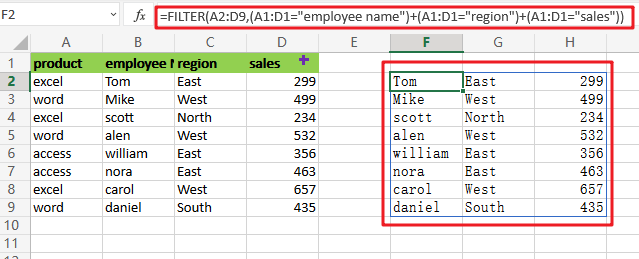So let’s get straight into it!

## General Formula

To filter out desired columns from the table, we would use the FILTER function and supply the horizontal array; the general formula is as follows:

`=FILTER(total_data,(range_name1=value1)+( range_name2=value2))`

As we have altered the above formula according to the example which we would discuss in this article to understand that how this formula works and how to use this formula:

`=FILTER(A2:D9,(A1:D1="employee name")+(A1:D1="region")+(A1:D1="sales"))`The end result is a filtered collection of data that only includes columns B, C, and D from the original data.

## Let’s See How This Formula Works:

Although FILTER is usually used to filter rows, it may also filter columns; the secret is to give an array with the same number of columns as the original data. In this example, we use boolean logic, commonly known as Boolean algebra, to create the necessary array.

Multiplication corresponds to AND logic in Boolean algebra and addition corresponds to OR logic. In the following example, we use Boolean algebra using OR logic (addition) to target only the columns “employee name”, “region”, and “sales” as follows:

After evaluating each expression, we obtain three arrays of TRUE/FALSE values:

`=(A1:D1="employee name")`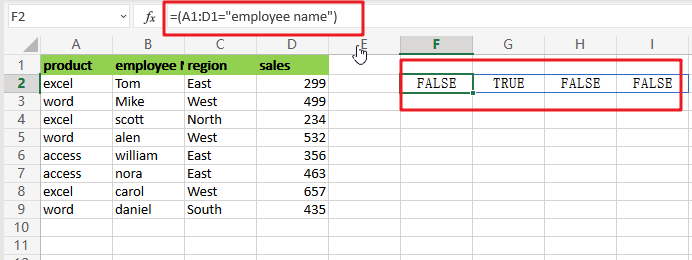`=(A1:D1="employee name")+(A1:D1="region")+(A1:D1="sales")`

The arithmetic action (addition) turns TRUE and FALSE values to 1s and 0s, so think of it like this: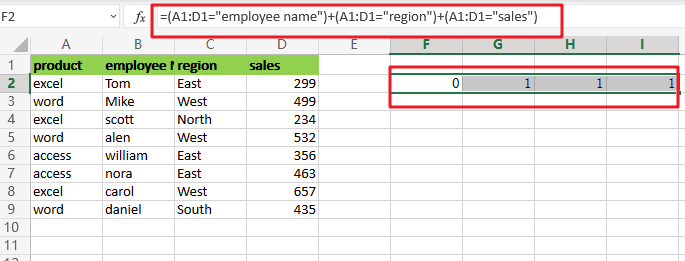This is sent straight to the FILTER function as the include argument:

`=FILTER(A2:D9,{0,1,1,1})`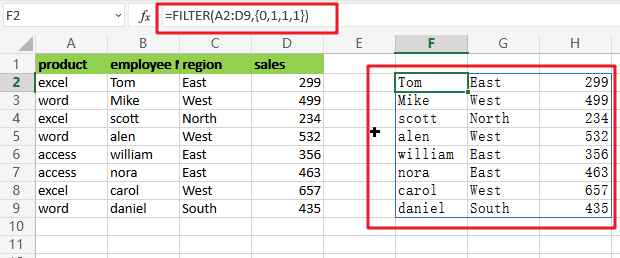In the source data, there are 4 columns and 4 values in the array, all of which are either 1 or 0. FILTER utilizes this array as a filter to include columns 2, 3, and 4 from the given data. Column  1 has been eliminated. In other words, the only columns that remain are those linked with 1s.

## Removing Columns Using the MATCH and Filter Functions

Applying OR logic with addition as demonstrated above works OK, but it does not scale well and makes it hard to utilize a range of numbers from a worksheet as the criterion. To create the include argument more quickly, you may use the MATCH function in conjunction with the ISNUMBER function as seen below:

`=FILTER(A2:D9,ISNUMBER(MATCH(A1:D1,{"employee name","region","sales"},0)))`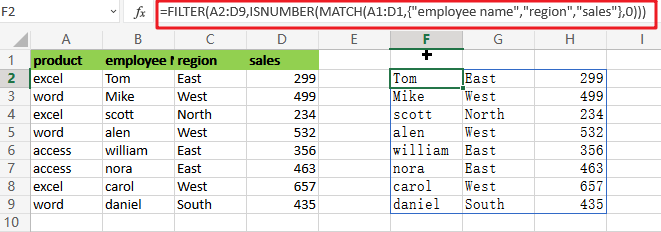`=MATCH(A1:D1,{"employee name","region","sales"},0)`

The MATCH function is set to seek for all column headings in the array constants ,”employee name“,”region” and “sales” as illustrated. We implement it this way so that the MATCH result has dimensions consistent with the original data, which has 4 columns. Also, the third parameter in MATCH is set to 0 to force an exact match.

MATCH provides an array that looks like this when it runs: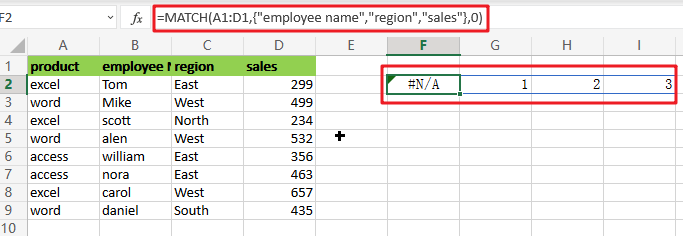`=ISNUMBER(MATCH(A1:D1,{"employee name","region","sales"},0))`

This array is sent straight to ISNUMBER, which produces another array: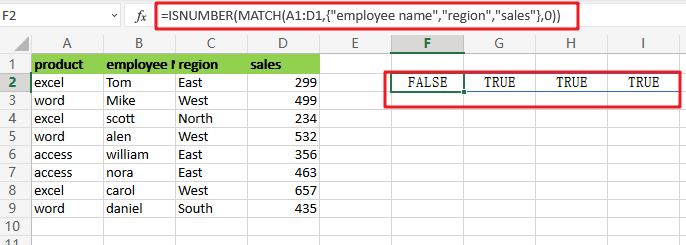Like the one above, this array is horizontal and has 4 values separated by commas. Column 1 is removed using the array by FILTER.

### Related Functions

• Excel ISNUMBER function
The Excel ISNUMBER function returns TRUE if the value in a cell is a numeric value, otherwise it will return FALSE.The syntax of the ISNUMBER function is as below:= ISNUMBER (value)…
• Excel Filter function
The FILTER function extracts matched records from a collection of data using one or more logical checks. The include argument specifies logical tests, which might encompass a wide variety of formula conditions.==FILTER(array,include,[if empty])…
• Excel MATCH  function
The Excel MATCH function search a value in an array and returns the position of that item.The MATCH function is a build-in function in Microsoft Excel and it is categorized as a Lookup and Reference Function.The syntax of the MATCH function is as below:= MATCH  (lookup_value, lookup_array, [match_type])….
Related Posts

Break ties with helper COUNTIF and column

Suppose you got a task to adjust the values that contain the ties; what would be your first attempt to break the ties of the given value? If you are wondering about doing this task manually, let me add that ...

Find the Closest Data to the Data Provided in Excel

In our daily work, we may encounter such an issue that to find the closest value to a certain value. In fact, Excel internal functions can help us solve this problem. In today’s article, we will show you how to ...

Abbreviate Names Or Words in Excel

As an MS Excel user, you might have come across a task where you need to abbreviate different names or words, and there are also possibilities that you might have done this task manually by assuming that there isn't any ...

Convert State Names To Abbreviations

Assume that you got a task to convert the full state's name into the abbreviations in MS Excel, and for doing this task, you might choose to do it manually, which is an acceptable way only if you don't have ...

Filter or Extract for Multiple OR Criteria

If you are an valid MS Excel user, you have probably come across a situation where you wanted to filter the data in a separate table with specific criteria. You could do this task manually, which is also acceptable when ...

Filter Multiple Values

In Excel, you can easily filter a table to display only the rows that meet your criteria. This is a quick way to find the information you need without scrolling through all the data. In this post, we'll show you ...

Excel XLOOKUP Function

Excel XLOOKUP Function was added into Excel as a beta feature in August 2019 and is now accessible exclusively in Microsoft 365. (as of July 2021). However, if you fall into this category and often deal with big sets of ...

Extract or Filter Top n values

You might have been through this kind of situation where you need to filter out the top n values from the list having few values, and I am also pretty sure about it that you might have chosen to do ...

Extract Unique Items From A List

This post will guide you how to extract unique itmes from a given list in Microsoft Excel. How to create a newly formula to get unique values from a range cells in Excel. The unique list of items is the ...

Extract all the matches with helper Column

With Excel's powerful functions IF, INDEX, and MATCH, we can find exactly what you're looking for with a few clicks of the mouse. This step-by-step tutorial will show how easy it is to extract data using these tools and more! ...

Sidebar